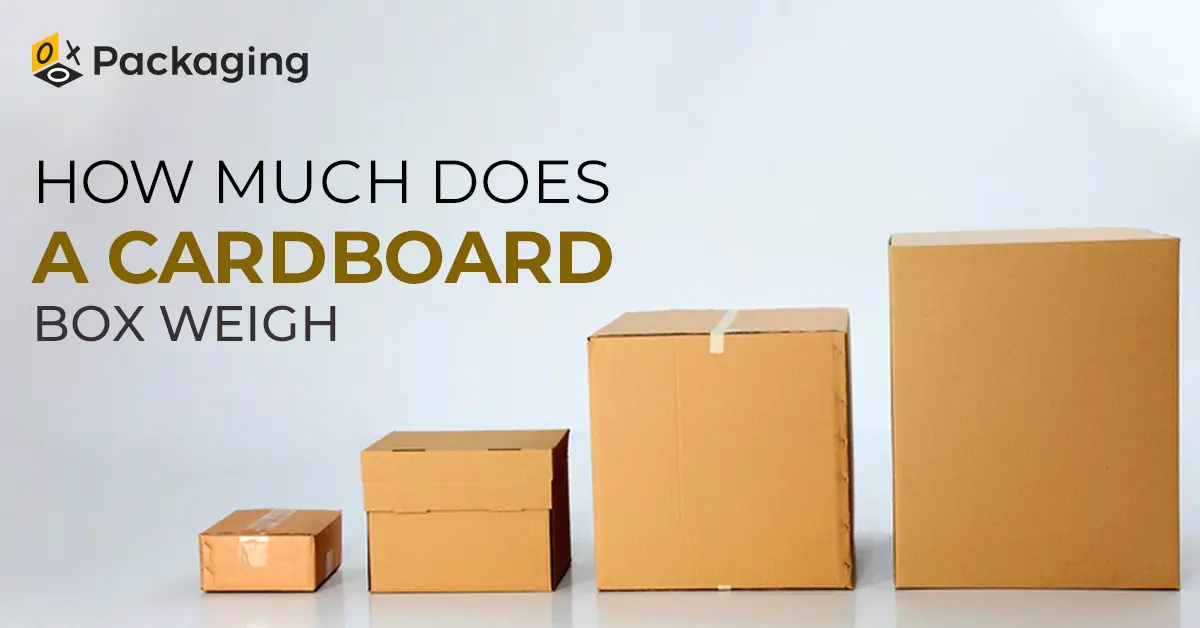(510) 500-9533|sales@oxopackaging.com

# How Much Does a Cardboard Box Weigh? Complete Guide

2023-08-09 10:27:44Cardboard is the most popular packaging material today due to its flexible nature and countless benefits. It can be cut into any shape, designed in any style, and printed with excellent visuals with high-end printing techniques. With its flexible nature, cardboard makes boxes for almost all kinds of products. Popularity brings questions, and when it comes to packaging, people have a lot of them. The most common is how much does a cardboard box weigh. So this blog will answer all your questions about cardboard boxes.

## How Much Does a Cardboard Box Weigh:

The weight of a cardboard box depends on a number of factors. The primary being its dimensions. How large or small a cardboard box is can help decide its weight.

The formula to calculate the weight of a cardboard box can be drawn by first multiplying the length, width, and height of the box. Once you have the figure, divide it by a number to get the weight. The said number is different according to the unit of weight you need. Let’s discuss the different units and the formulas to calculate the weight for each unit.

• ### How Much Does A Cardboard Box Weigh In Pounds?

To calculate the weight of a cardboard box in pounds, you need to divide the box dimensions by 166. The number 166 is the assumed density per cubic foot of the cardboard used to make the box. Using this formula, say you want to know how much does a 12x12x12 cardboard box weigh. So this can be calculated by;
Weight = (12x12x12)/166
Weight = 1728/166
Weight = 10.4 Pounds
So the approximate weight of a 12x12x12 inches box would be around 10.4 pounds.

• ### How Much Does A Cardboard Box Weigh In Ounces?

The weight of a cardboard box can be converted to ounces by using the weight in pounds. Just divide the weight in pounds by 16 to get the weight in ounces. So for this box, you can do the following;
Weight = 10.4 Pounds / 16
Weight = 0.665 Ounces
This can also help you figure out how much does a small cardboard box weigh in oz.

• ### How Much Does A Cardboard Box Weigh In KG?

To calculate the weight of a cardboard box in KG, we divide the volume in cm3 by 6000. The reason for dividing by 6000 is to convert from cubic centimeters to kilograms. To calculate the weight of the same box in kilograms, we first have to convert the values of the length, width, and height from inches to centimeters to get the volume in cubic centimeters.
Length, Width, Height = 12 x 2.54 cm/inch
= 30.48 cm
Now that we have the dimensions in centimeters, we can calculate the weight.
Weight = (30.48cm x 30.48cm x 30.48cm)/6000
Weight = 28316.8466 cm³ / 6000
Weight = 4.7194 kilograms

• ### How Much Does A Cardboard Box Weigh In Grams?

Calculating the weight of a cardboard box in grams is relatively easier. You can just convert the weight in kilograms to grams by multiplying by 1000. So the weight of the same box in grams would be;
Weight = 4.7194 x 1000
Weight = 4719.4 grams
So if you want to know how much does a small cardboard box weigh in grams or even want to know the weight of a larger box, this calculation can help.

## How Much Does A Large Cardboard Box Weigh?

The above formulas can be used to calculate the weight of any size of a cardboard box. Even if you want to know how much does a small cardboard box weighs or the weight of a larger box, you can use these dimensions to achieve that.

However, remember that these calculations offer only an approximation of the weight of the cardboard box based on dimensions. The exact weight may also depend on the thickness of the material, along with other factors. So it is advised to use a scale to measure the exact weight of a cardboard box.

Read More: How to Measure Box Dimension

## How Much Does A Pallet Of Cardboard Boxes Weigh?

The weight of a pallet of cardboard boxes depends on many factors. These may include the number of boxes in a pallet, the weight of individual units, the size of the cardboard boxes, and much more. On average, a pallet of cardboard boxes has 60 boxes and weighs anywhere around 30 - 50 pounds.

The size of the cardboard boxes has a lot to do with the weight of the pallet. For example, if you have a pallet with 50 medium-sized cardboard boxes, each weighing 10 pounds, the weight of the pallet would be 500 pounds. But if you have the same number of large boxes, each weighing around 15 pounds, the weight of the pallet would be 750 pounds. So how much does a cardboard box weigh can hugely affect the weight of the pallet.

## Conclusion:

This blog explains everything you need to know about calculating the weight of a cardboard box. Cardboard is an amazing material and is the perfect choice for packaging boxes. If you have any other questions regarding cardboard boxes, you can reach out. Keep visiting OXO Packaging for custom packaging boxes, packaging related news and information.

×